# JP6295650B2 - Audio signal processing apparatus and program - Google Patents

## Info

Publication number
JP6295650B2
JP6295650B2 JP2013267711A JP2013267711A JP6295650B2 JP 6295650 B2 JP6295650 B2 JP 6295650B2 JP 2013267711 A JP2013267711 A JP 2013267711A JP 2013267711 A JP2013267711 A JP 2013267711A JP 6295650 B2 JP6295650 B2 JP 6295650B2
Authority
JP
Japan
Prior art keywords
coherence
filter coefficient
arrival direction
coherence filter
microphones
Prior art date
Legal status (The legal status is an assumption and is not a legal conclusion. Google has not performed a legal analysis and makes no representation as to the accuracy of the status listed.)
Active
Application number
JP2013267711A
Other languages
Japanese (ja)
Other versions
JP2015126279A (en
Inventor

Original Assignee

Priority date (The priority date is an assumption and is not a legal conclusion. Google has not performed a legal analysis and makes no representation as to the accuracy of the date listed.)
Filing date
Publication date
Application filed by 沖電気工業株式会社 filed Critical 沖電気工業株式会社
Priority to JP2013267711A priority Critical patent/JP6295650B2/en
Publication of JP2015126279A publication Critical patent/JP2015126279A/en
Application granted granted Critical
Publication of JP6295650B2 publication Critical patent/JP6295650B2/en
Application status is Active legal-status Critical
Anticipated expiration legal-status Critical

## Images

•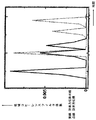•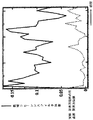•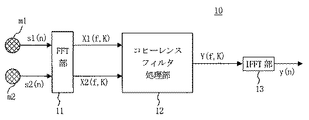•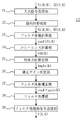•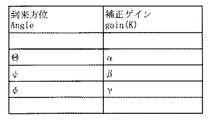•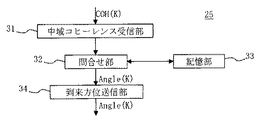••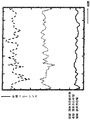•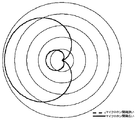•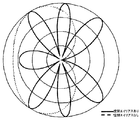•## Description

The present invention relates to an audio signal processing apparatus and program, and handles, for example, an audio signal from a telephone or a video conference apparatus (in this specification, an audio signal such as an audio signal or an acoustic signal is called an “audio signal”). It can be applied to communication devices and communication software.

One of the methods for suppressing the noise component contained in the acquired audio signal is a coherence filter method. As described in Patent Document 1, the coherence filter method is a method of suppressing a noise component having a large bias in the arrival direction by multiplying the cross-correlation of signals having blind spots on the left and right for each frequency.

In the audio signal processing apparatus to which the coherence filter method is applied, the first directional signal including only the component arriving from the left side of the front and the component arriving from the right side using the input signals obtained by the two microphones. The second directional signal including only the first directional signal is generated, and the noise value is suppressed by multiplying the input signal as a coefficient value by the correlation value (coherence filter coefficient) for each frequency component of the first and second directional signals. Getting the signal. Here, an arithmetic expression of the coherence filter coefficient is described in Patent Document 1.

Here, the coherence that is the average value of the coherence filter coefficients for each frequency component that is the correlation value of the first and second directional signals is the disturbing sound (sound or sound that interferes with the sound to be extracted). It is a parameter that reflects the direction of arrival. Patent Document 2 describes that coherence in a disturbing sound section is used for estimating the arrival direction of the disturbing sound.

JP2013-126026A JP2013-182044

Asano Tadashi, edited by the Acoustical Society of Japan, "Sound Array Signal Processing", Corona, first published in February 2011

An audio signal processing device to which the coherence filter method is applied is applied to, for example, an audio input / recognition device of a smartphone. It may be possible to provide two microphones with a distance (for example, about 14 cm) in the longitudinal direction of the smartphone. In this case, the distance between the two microphones (hereinafter referred to as “distance” (for example, about 3 cm) with respect to the width direction of the smartphone) , Referred to as the microphone spacing) is quite large.

When the distance between the microphones exceeds a predetermined value, the directivity of the low frequency component of the first and second directional signals increases. FIG. 9 is an explanatory diagram of this. FIG. 9 shows the directivity for a certain low frequency component (for example, about 500 Hz) in the first directivity signal. The broken line curve represents the case where the microphone interval is narrow, and the solid line curve represents the case where the microphone interval is wide. The directivity of the direction shifted 90 ° to the right from the front is several times as the microphone interval is wide (4 in FIG. 9). Doubled) The directivity is sharp. Note that the circle in FIG. 9 is drawn as a scale that gives a measure of the intensity of directivity.

When the coherence filter coefficient is calculated based on the first and second directivity signals having sharp directivity, the behavior of the coherence filter coefficient is greatly changed as compared with the case where the microphone interval is narrow. This is because, for example, when the interfering sound arrives from the left, the first directional signal falls in the blind spot and the signal value becomes small. This is because it is included in the above. Therefore, since the characteristic difference between the first and second directional signals becomes extremely large, the correlation (coherence filter coefficient) becomes extremely small. When such a coherence filter coefficient is applied to noise suppression processing, a strong noise suppression effect can be obtained, but the target speech component is greatly distorted, so that the sound quality is significantly degraded.

In addition, when the distance between the microphones is increased, there is a problem that a kind of error component called a spatial alias is mixed and the shape of the directivity to be formed is deformed (see page 76 of Non-Patent Document 1). FIG. 10 shows an example of a change in directivity due to a spatial alias. FIG. 10 shows the directivity at a certain frequency in the first directivity signal. The dotted curve shows the directivity when the microphone interval is narrow, and has a cardioid shape. On the other hand, the solid curve shows the directivity when the distance between the microphones is wide, and exhibits a unique shape having blind spots in various directions due to the spatial alias.

When the coherence filter coefficient is calculated from the first and second directivity signals having such directivity and further the coherence is calculated from the coherence filter coefficient, the behavior of the coherence changes greatly. This is because the directivity has a shape that is nearly symmetrical, and if the arrival direction of noise is, for example, 45 ° diagonally to the left, both the first and second directional signals capture the interference sound. For this reason, the correlation is increased in spite of the interference sound, and as a result, the coherence is increased.

As described above, when the microphone interval becomes large and a spatial alias component is mixed, there is a problem that an accurate estimation result cannot be obtained in the determination of the arrival direction.

Therefore, an audio signal processing apparatus and program that can obtain a sufficient audio processing result even when the microphone interval is large is desired.

According to a first aspect of the present invention, there is provided an audio signal processing device that suppresses noise components included in an input audio signal obtained by a pair of microphones by coherence filter processing. (1) Coherence filter for calculating coherence filter coefficients Coefficient calculation means, (2) arrival direction estimation means for estimating the arrival direction of the disturbing sound included in the input voice signal, and (3) obtaining a correction gain corresponding to the estimated arrival direction, A low-pass filter coefficient correction unit that corrects the coherence filter coefficient of the signal with the correction gain; and (4) a filter that performs coherence filter processing by applying the coherence filter coefficient of the entire band including the corrected low-frequency coherence filter coefficient. possess a process execution means, (5) the correction gain corresponding to the arrival direction, the distance between the pair of microphones Characterized in that it is determined based on the ratio of the coherence filter coefficient when the distance between the pair of microphones is wide with respect to coherence filter coefficients a narrow case.

The second aspect of the present invention is an audio signal processing program that suppresses noise components contained in an input audio signal obtained by a pair of microphones by coherence filter processing, comprising: (1) a coherence filter coefficient; A coherence filter coefficient calculating means for calculating (2) an arrival direction estimating means for estimating the arrival direction of the disturbing sound included in the input voice signal, and (3) a correction gain corresponding to the estimated arrival direction. And a low-pass filter coefficient correction means for correcting the low-frequency coherence filter coefficient with the correction gain, and (4) applying a coherence filter coefficient of the entire band including the corrected low-frequency coherence filter coefficient. the correction gain filtering to function as a filter processing performing means for performing, corresponding to arrival direction (5) And characterized in that it is determined based on the ratio of the coherence filter coefficient when the distance between the pair of microphones is wide with respect to coherence filter coefficient when the distance between the pair of microphones is narrow.

According to a third aspect of the present invention, there is provided an audio signal processing apparatus for estimating an arrival direction of an interfering sound included in an input audio signal acquired by a pair of microphones. (1) calculating at least a coherence filter coefficient in a limited band Coherence filter coefficient calculation means for performing, (2) limited band coherence calculation means for calculating the limited band coherence by applying the limited band coherence filter coefficient, and (3) various values and arrival directions of the limited band coherence. Are obtained in advance, and based on the calculated coherence of the limited band, the arrival direction obtaining means for obtaining the associated arrival direction and outputting it as an estimated value , and (4) correction corresponding to the estimated arrival direction to obtain a gain, closed and limited band filter coefficient correcting means for correcting the coherence filter coefficients of limited band in the correction gain , That it is determined based on the correction gain, the ratio of coherence filter coefficient when the distance between the pair of microphones for coherence filter coefficient when the distance between the pair of microphones narrow wide corresponding to arrival direction (5) Features.

A fourth aspect of the present invention is an audio signal processing program for estimating an arrival direction of an interfering sound included in an input audio signal acquired by a pair of microphones, and comprising: (1) at least a limited band; A coherence filter coefficient calculating means for calculating a coherence filter coefficient; (2) a limited band coherence calculating means for calculating a limited band coherence by applying a limited band coherence filter coefficient; and (3) various types of limited band coherence. An arrival direction acquisition means that associates a value with an arrival direction in advance, obtains the corresponding arrival direction based on the calculated coherence of the limited band, and outputs it as an estimated value; and (4) estimated arrival Obtain a correction gain corresponding to the direction and correct the limited band coherence filter coefficient with the correction gain. A data coefficient correcting means is functioning, (5) the correction gain corresponding to the arrival direction, the ratio of the coherence filter coefficient when the distance between the pair of microphones for coherence filter coefficient when the distance between the pair of microphones is narrow wide It is determined based on .

According to the present invention, it is possible to realize an audio signal processing apparatus and program that can obtain a sufficient audio processing result even when the microphone interval is large.

It is explanatory drawing which shows the behavior of the coherence filter coefficient in the low region when the microphone interval is wide for two arrival directions. It is explanatory drawing which shows the behavior of the coherence filter coefficient in the low region when the microphone interval is narrow for two arrival directions. It is a block diagram which shows the whole structure of the audio | voice signal processing apparatus of 1st Embodiment. It is a block diagram which shows the detailed structure of the coherence filter process part in the audio | voice signal processing apparatus of 1st Embodiment. It is explanatory drawing which shows the conversion table which matched the arrival direction and the correction gain which the correction gain determination part in the audio | voice signal processing apparatus of 1st Embodiment utilizes. It is a block diagram which shows the detailed structure of the arrival direction estimation part in the coherence filter process part in the audio | voice signal processing apparatus of 1st Embodiment. It is explanatory drawing which shows the conversion table which matched the mid range coherence and the arrival direction which the arrival direction estimation part in the coherence filter process part in the audio | voice signal processing apparatus of 1st Embodiment uses. It is explanatory drawing which shows the behavior of the mid-range coherence for every arrival direction of disturbance sound. It is explanatory drawing of the sharpness of the directivity of the low frequency component in a directivity signal increasing when a microphone space | interval spreads beyond a predetermined value. It is explanatory drawing which shows the example of the change of the directional characteristic by a space alias. It is explanatory drawing of the term showing an angle.

(A) First Embodiment Hereinafter, a first embodiment of an audio signal processing device and a program according to the present invention will be described in detail with reference to the drawings. The speech processing apparatus according to the first embodiment applies a coherence filter method to suppress a noise component contained in a speech signal.

(A-1) Concept of the First Embodiment As described above, the phenomenon that the directivity of the low frequency component in the directional signal becomes strong accompanying the increase in the microphone interval is unavoidable. When the microphone interval is wide enough to increase the directivity of the low frequency component, the sound quality and the suppression performance are moderately compatible in the first embodiment so that excessive suppression processing is not performed. The coherence filter coefficient was corrected to the value.

FIG. 1 and FIG. 2 are diagrams comparing the behavior of coherence filter coefficients in the low band when the microphone interval is wide and when the microphone interval is narrow, with respect to two arrival azimuths (diagonally forward and lateral), and FIG. 1 shows a wide microphone interval. FIG. 2 shows the behavior when the microphone interval is narrow. In this specification, “oblique front”, “middle” and “horizontal” described later refer to directions shifted by an angle as shown in FIG. 11 with respect to the front (front). When the microphone interval is wide, as can be seen from FIG. 1, the coherence filter coefficient takes a very small value and hardly changes due to the arrival direction of the interference sound. On the other hand, when the microphone interval is narrow, as can be seen from FIG. 2, the coherence filter coefficient increases, and the difference in the range depending on the arrival direction becomes significant.

Based on such characteristics, when the microphone interval is wide, the coherence filter coefficient value is moderately compatible with the suppression performance and sound quality when the microphone interval is narrow. Specifically, for each arrival direction, the coherence ratio between the coefficient value when the microphone interval is narrow and the microphone interval is calculated and stored in advance, and this is corrected to the low-frequency coherence filter coefficient. Multiply as gain. As described above, since the range of the low-frequency coherence filter coefficient can be specified to some extent when the microphone interval is narrow and wide, it is possible to determine the ratio of both in advance.

The first embodiment is a case where the microphone interval is wide (for example, a few tens of centimeters), and the first embodiment corrects the low-frequency coherence filter coefficient, thereby reducing the coherence filter coefficient when the microphone interval is narrow. It tries to improve the sound quality close to the characteristics.

(A-2) Configuration of First Embodiment FIG. 3 is a block diagram illustrating a configuration of an audio signal processing device according to the first embodiment. Here, the part excluding the pair of microphones m1 and m2 can be configured by hardware, and can also be realized by software (audio signal processing program) executed by the CPU and the CPU. However, even if any implementation method is adopted, it can be functionally represented in FIG.

3, the audio signal processing apparatus 10 according to the first embodiment includes a pair of microphones m1 and m2, an FFT (Fast Fourier Transform) unit 11, a coherence filter processing unit 12, and an IFFT (Inverse Fast Fourier Transform) unit 13. Have.

The pair of microphones m1 and m2 are arranged apart from each other by a predetermined distance (or an arbitrary distance) wide enough to cause the above-described problem, and each captures surrounding sounds. Each of the microphones m1 and m2 is omnidirectional (or has a very gentle directivity in the front direction). Audio signals (input signals) captured by the respective microphones m1 and m2 are converted into digital signals s1 (n) and s2 (n) via corresponding A / D converters (not shown) and given to the FFT unit 11. . Note that n is an index indicating the input order of samples, and is expressed as a positive integer. In the text, it is assumed that the smaller n is the older input sample, and the larger n is the newer input sample. The band of the audio signal (input signal) is, for example, 0 Hz to 8000 Hz. There are a low band and a middle band described later as partial bands in this band.

The FFT unit 11 receives input signal sequences s1 (n) and s2 (n) from the microphones m1 and m2, and performs fast Fourier transform (or discrete Fourier transform) on the input signals s1 and s2. Thereby, the input signals s1 and s2 can be expressed in the frequency domain. In performing the Fast Fourier Transform, analysis frames FRAME1 (K) and FRAME2 (K) composed of predetermined N samples are configured and applied from the input signals s1 (n) and s2 (n). An example of constructing the analysis frame FRAME1 (K) from the input signal s1 (n) is shown in the following equation (1), and the analysis frame FRAME2 (K) is the same.

K is an index indicating the order of frames and is expressed by a positive integer. In the text, it is assumed that the smaller the K, the older the analysis frame, and the larger, the newer the analysis frame. In the following description, it is assumed that the index representing the latest analysis frame to be analyzed is K unless otherwise specified.

The FFT unit 11 converts the frequency domain signals X1 (f, K) and X2 (f, K) into the frequency domain signals X1 (f, K) by performing a fast Fourier transform process for each analysis frame. And X2 (f, K) are supplied to the coherence filter processing unit 12, respectively. Note that f is an index representing a frequency. X1 (f, K) is not a single value, but is composed of spectral components of a plurality of frequencies f1 to fm, as shown in equation (2). Furthermore, X1 (f, K) is a complex number and consists of a real part and an imaginary part. The same applies to X2 (f, K) and later-described B1 (f, K) and B2 (f, K).

X1 (f, K) = {X1 (f1, K), X1 (f2, K),..., X1 (fm, K)} (2)
In the coherence filter processing unit 12 to be described later, the frequency domain signal X1 (f, K) of the frequency domain signals X1 (f, K) and X2 (f, K) is mainly used, and the frequency domain signal X2 (f, K) is used. ) As a sub (see equation (7) described later), however, the frequency domain signal X2 (f, K) may be the main and the frequency domain signal X1 (f, K) may be the sub.

The coherence filter processing unit 12 has a detailed configuration shown in FIG. 4 to be described later, performs coherence filter processing, obtains a signal Y (f, K) in which a noise component is suppressed, and gives it to the IFFT unit 13. is there.

The IFFT unit 13 performs an inverse fast Fourier transform on the noise-suppressed signal Y (f, K) to obtain an output signal y (n) that is a time domain signal.

FIG. 4 is a block diagram illustrating a detailed configuration of the coherence filter processing unit 12.

In FIG. 4, the coherence filter processing unit 12 includes an input signal receiving unit 21, a directivity forming unit 22, a filter coefficient calculation unit 23, a coherence calculation unit 24, an arrival direction estimation unit 25, a correction gain determination unit 26, and a filter coefficient correction unit. 27, a filter processing unit 28 and a filtered signal transmission unit 29.

The input signal receiving unit 21 receives the frequency domain signals X1 (f, K) and X2 (f, K) output from the FFT unit 11.

The directivity forming unit 22 forms the first and second directivity signals B1 (f, K) and B2 (f, K). As a method of forming the directivity signals B1 (f, K) and B2 (f, K), an existing method can be applied. For example, a method of obtaining by calculation according to the equations (3) and (4). Can be applied.

The filter coefficient calculation unit 23 calculates the coherence filter coefficient coef (f, K) according to the equation (5) based on the first and second directivity signals B1 (f, K) and B2 (f, K). To do.

The coherence calculating unit 24 calculates coherence COH (K) as an index value that can estimate the arrival direction of the disturbing sound based on the coherence filter coefficient coef (f, K) according to the equation (6). The coherence COH (K) is obtained by arithmetically averaging the coherence filter coefficient coef (f, K) at a middle frequency (for example, about 2000 Hz to 4000 Hz as a middle frequency) or at all frequencies as shown in the equation (6). Value.

The arrival direction estimation unit 25 estimates the arrival direction of the disturbing sound and obtains the estimated arrival direction Angle. Here, the arrival azimuth refers to an angle deviated from the front azimuth. For example, when Θ is deviated to the right side or Θ is deviated to the left side, it is similarly Θ, and information on which side is deviated is not included. As will be described later, in determining the correction gain gain (K), information on which side is shifted is not necessary.

Although the arrival direction estimation unit 25 may perform estimation without using coherence COH (K), a case will be described below in which estimation is performed using coherence COH (K). The arrival direction estimation unit 25 may apply an existing method as described in Patent Literature 2 as an arrival direction estimation method using coherence COH (K). However, in the first embodiment, estimation is performed according to an estimation method using a new coherence COH (K) executed by a detailed configuration shown in FIG. When the existing estimation method as described in Patent Document 2 is applied, the above-described coherence calculation unit 24 calculates the coherence COH (coherence COH () as a value obtained by arithmetically averaging the coherence filter coefficients coef (f, K) at all frequencies. K) is calculated. On the other hand, when the estimation method applied by the detailed configuration shown in FIG. 6 is applied, the coherence calculation unit 24 described above is an arithmetic average value of the coherence filter coefficients coef (f, K) for the frequencies in the middle range. To calculate coherence COH (K).

The correction gain determination unit 26 obtains a correction gain gain (K) for the low frequency (for example, 1000 Hz or less) coherence filter coefficient coef (f, K) based on the estimated arrival direction Angle. An example of the correction gain determination unit 26 is one that uses a table shown in FIG. 5 in which the estimated arrival direction Angle and the correction gain gain (K) are associated with each other. FIG. 5 shows that when the estimated arrival direction Angle belongs to the range Θ, the correction gain gain (K) is α, and when the estimated arrival direction Angle belongs to the range ψ, the correction gain gain (K) is β, and the estimated arrival direction Angle Represents that the correction gain gain (K) is set to γ when belongs to the range φ. In the correction gain gain (K), a ratio of the coherence filter coefficient when the microphone interval is narrow to the coherence filter coefficient when the microphone interval is wide in the arrival direction is set, and the correction gain gain (K) is set as the microphone. By multiplying the coherence filter coefficient when the interval is wide, the coefficient can be converted into the coherence filter coefficient when the microphone interval is narrow.

If the arrival direction estimation unit 25 can estimate only by a value shifted by a predetermined angle such as diagonally forward, intermediate (intermediate angle between diagonally forward and horizontal), and horizontal, the estimated arrival direction Angle of the table includes Describe the estimated angle. Further, as the correction gain determination unit 26, a function using a function for calculating the correction gain gain (K) from the estimated arrival direction Angle may be applied.

The filter coefficient correction unit 27 corrects the low-frequency coherence filter coefficient coef (f, K) by a correction gain gain (K) times.

The filter processing unit 28 applies the coherence filter coefficient coef (f, K) after the low frequency correction, and performs the coherence filter process on the main frequency domain signal X1 (f, K) as shown in the equation (7). To obtain a noise-suppressed signal (filtered signal) Y (f, K). In addition, (7) Formula represents each calculation (multiplication process) of each frequency.

Y (f, K) = X1 (f, K) × coef (f, K) (7)
Here, the physical meaning of the coherence filter process will be supplemented. The coherence filter coefficient coef (f, K) (the same applies to the coherence filter coefficient after low-frequency correction) is a cross-correlation of signal components having blind spots on the left and right. It is a voice component coming from the front, and when the correlation is small, the arrival direction is also a component that is biased to the right or left. Therefore, multiplication by the coherence filter coefficient coef (f, K) can be said to be processing for suppressing a noise component coming from the side.

The post-filter processing signal transmission unit 29 supplies the post-noise suppression signal Y (f, K) to the IFFT unit 13 at the subsequent stage. Further, the post-filter processing signal transmission unit 29 increases K by 1 and starts processing of the next frame.

FIG. 6 is a block diagram showing a detailed configuration of the arrival direction estimation unit 25 described above. In FIG. 6, the arrival direction estimation unit 25 includes a coherence reception unit 31, an inquiry unit 32, a storage unit 33, and an arrival direction transmission unit 34.

The coherence receiver 31 receives the coherence COH (K) calculated by the coherence calculator 24. The coherence COH (K) here is a value obtained by arithmetically averaging the coherence filter coefficients coef (f, K) for the middle frequency (or its frequency), and may be hereinafter referred to as middle frequency coherence.

The storage unit 33 stores a conversion table in which the mid-range coherence COH (K) and the arrival direction Angle are associated with each other.

The inquiry unit 32 extracts the arrival direction Angle corresponding to the mid-range coherence COH (K) received by the coherence receiving unit 31.

The arrival direction transmission unit 34 outputs the arrival direction Angle extracted by the inquiry unit 32 to the correction gain determination unit 26.

FIG. 7 is an explanatory diagram of the description content of the conversion table in the storage unit 33. In the example of FIG. 7, when the mid-range coherence COH (K) is greater than or equal to A and less than B, the diagonally preceding direction is associated as the arrival azimuth Angle, and when the mid-range coherence COH (K) is greater than or equal to B and less than C, the arrival azimuth Angle And the middle as the arrival direction Angle when the mid-range coherence COH (K) is C or more and less than D.

This conversion table is configured so that the arrival direction can be obtained based on the magnitude relationship peculiar to the mid-range coherence filter coefficient including the spatial alias.

FIG. 8 is an explanatory diagram illustrating the behavior of the mid-range coherence for each arrival direction of the disturbing sound. In the middle frequency band of 2000 Hz to 4000 Hz, as shown in FIG. 10 described above, the shape has directivity in several directions and is nearly symmetrical. Therefore, a difference in the mid-range coherence COH (K) depending on the arrival direction is likely to occur, such that the mid-range coherence COH (K) is small in one arrival direction and the mid-range coherence COH (K) is large in another direction. Based on this point, the conversion table of FIG. 7 is configured. It should be noted here that the range of the mid-range coherence according to the arrival direction is such that the magnitude function of the mid-range coherence COH (K) and the arrival direction are expressed as in the case of diagonally forward <the side case <the middle case]. There is no relationship between the magnitude relationship and the monotone increasing function or the monotonic decreasing function. Despite the absence of such a monotonous relationship, the inventors found out that the arrival direction can be estimated using the mid-range coherence COH (K) because of the originality of the present inventors.

(A-3) Operation of the First Embodiment Next, the operation of the audio signal processing device 10 of the first embodiment will be described with reference to the drawings, the entire operation, the detailed operation in the coherence filter processing unit 12, and the arrival direction. The detailed operation in the estimation unit 25 will be described in order.

Signals s1 (n) and s2 (n) input from the pair of microphones m1 and m2 are respectively converted from time domain to frequency domain signals X1 (f, K) and X2 (f, K) by the FFT unit 11. Is then provided to the coherence filter processing unit 12. Thus, the coherence filter processing unit 12 performs coherence filter processing, and the obtained noise-suppressed signal Y (f, K) is provided to the IFFT unit 13. In IFFT section 13, noise-suppressed signal Y (f, K), which is a frequency domain signal, is converted into time domain signal y (n) by inverse fast Fourier transform, and this time domain signal y (n) is output. Is done.

Next, a detailed operation in the coherence filter processing unit 12 will be described. The above-described FIG. 4 showing the detailed configuration of the coherence filter processing unit 12 can also be regarded as a flowchart showing the processing of the coherence filter processing unit 12. Hereinafter, processing of a certain frame will be described, but the processing described below is repeated for each frame.

When it becomes a new frame and the frequency domain signals X1 (f, K) and X2 (f, K) of the new frame (current frame K) are given from the FFT unit 11, according to the equations (3) and (4) , First and second directional signals B1 (f, K) and B2 (f, K) are calculated, and based on these directional signals B1 (f, K) and B2 (f, K), The coherence filter coefficient coef (f, K) is calculated according to the equation (5). Furthermore, based on the coherence filter coefficient coef (f, K), the mid-range coherence COH (K) is calculated as an index value that can estimate the arrival direction of the disturbing sound according to the equation (6).

Thereafter, the arrival direction estimation unit 25 refers to the conversion table shown in FIG. 7 using the mid-range coherence COH (K) as a key to estimate the arrival direction Angle of the disturbing sound.

Then, the correction gain determination unit 26 refers to the conversion table of FIG. 5 using the estimated arrival direction Angle as a key, and obtains a correction gain gain (K) for the low frequency coherence filter coefficient coef (f, K). In the coefficient correction unit 27, the low-frequency coherence filter coefficient coef (f, K) is corrected by being multiplied by the correction gain gain (K).

Thereafter, the filter processing unit 28 performs the coherence filter process on the main frequency domain signal X1 (f, K) according to the equation (7) based on the obtained low frequency corrected coherence filter coefficient coef (f, K). The obtained noise-suppressed signal (filtered signal) Y (f, K) is provided to the IFFT unit 13 and the frame variable K is incremented by 1, and the process proceeds to the next frame.

Next, a detailed operation in the arrival direction estimation unit 25 will be described.

When the coherence receiving unit 31 is given the mid-range coherence COH (K) calculated by the coherence calculation unit 24, the interrogation unit 32 of the arrival direction estimation unit 25 gives the given mid-range coherence COH (K The arrival direction Angle corresponding to () is taken out and output.

The above is the outline of the operation of the first embodiment.

(A-4) Effects of the First Embodiment As described above, according to the first embodiment, the microphone can be used even when the directivity formed in the low frequency band becomes extremely strong due to an increase in the interval between the microphones. Based on the difference in behavior peculiar to the coherence filter coefficient when the interval is narrow and the coherence filter factor when the interval is wide, the coherence filter coefficient can be corrected to a value close to the coherence filter factor when the interval is small. As a result, the sound quality does not deteriorate due to excessive suppression processing. In addition, the restriction on the microphone interval is relaxed, and the designer can configure the microphone array at an arbitrary interval.

Further, according to the first embodiment, it is possible to estimate the arrival direction based on the behavior peculiar to the mid-range coherence filter coefficient even when the space between the microphones is widened and a spatial alias is mixed. Thereby, the restriction on the microphone interval is relaxed, and the designer can configure the microphone array at an arbitrary interval.

Due to the above effects, it is possible to expect improvement in call sound quality in a communication device such as a video conference device, a mobile phone, or a smartphone to which the audio signal processing device or program of the first embodiment is applied.

(B) Other Embodiments Also in the description of the first embodiment, various modified embodiments have been mentioned, and further modified embodiments as exemplified below can be given.

In the first embodiment, the low-frequency coherence filter coefficient is corrected with the correction gain. However, in addition to the correction gain, an adjustment coefficient may be multiplied to adjust the noise suppression performance and sound quality. For example, the adjustment coefficient may be changed by an operation on an adjustment operator (a predetermined key on the keyboard may be used), or only whether or not the adjustment coefficient is applied may be specified. good.

In the first embodiment, the distance between the pair of microphones m1 and m2 is fixed. However, at least one of the microphones may be movable and the distance between the microphones m1 and m2 may be variable. In this case, the conversion table to be applied may be switched according to the microphone interval. For example, a plurality of conversion tables such as a conversion table for a microphone interval of 8 cm to 10 cm, a conversion table for a microphone interval of 10 cm to 12 cm, and a conversion table for a microphone interval of 12 cm to 14 cm are prepared. A conversion table to be applied may be selected according to the interval. Here, the microphone interval may be input by the user, the microphone moving position may be provided stepwise, and the microphone interval may be automatically obtained by a sensor provided at each step.

In the first embodiment, the case where the arrival direction Angle is obtained using the mid-range coherence COH (K) as a key and the correction gain gain (K) is obtained using the arrival direction Angle as a key has been described. And a correction table that directly associates the correction gain gain (K) with each other, and the correction gain gain (K) may be directly obtained using the mid-range coherence COH (K) as a key. In the case of applying the other arrival direction estimation methods described in the first embodiment, similar modified embodiments can be cited.

In the first embodiment, the correction gain is obtained from the estimated arrival direction. However, in addition to this, the estimated arrival direction may be applied for other purposes. For example, a flooring threshold determined according to the direction of arrival of the disturbing sound may be applied to apply a flooring process to the high frequency coherence filter coefficient (Japanese Patent Application No. 2013-154825 and drawings).

In the first embodiment, the low frequency to be corrected by applying the correction gain is the same in any arrival azimuth. However, the width of the low frequency to be corrected is changed depending on the arrival azimuth. May be. For example, when the arrival direction is X, the low range may be up to 1000 Hz, and when the arrival direction is Y, the low range may be up to 1100 Hz. In addition to this, or in place of this, the width of the low band may be changed by the microphone interval.

In the first embodiment, the same midrange is shown regardless of the microphone interval. However, at least one of the width of the midrange and the center frequency of the midrange may be changed depending on the microphone interval.

The use of the method of estimating the arrival direction described in the first embodiment is not limited to noise suppression according to the coherence filter method, but various signal processing that requires information on the arrival direction of the disturbing sound. Can be applied to. For example, the arrival direction estimation method described in the first embodiment can be applied to noise suppression processing other than the coherence filter method, sound source separation processing, speech encoding processing, and the like.

Depending on the application, the calculation itself of the coherence filter coefficient may be limited to the middle range.

Here, in the method of estimating the arrival direction described in the first embodiment, the arrival direction is estimated as the deviation angle (absolute value) from the front. However, the arrival direction is from the right side or from the left side. If such information is required, the calculation of equation (8) is performed. If the obtained value is positive, the arrival from the right side is determined. If the obtained value is negative, the arrival from the left side is determined. Anyway.

In the first embodiment, the processing that has been processed with the frequency domain signal may be performed with the time domain signal, if possible. If possible, processing may be performed with a frequency domain signal.

In the first embodiment, the coherence filter method is applied alone as the noise suppression technique. However, the noise suppression technique is used in combination with other noise suppression techniques such as the voice switch method, the Wiener filter method, and the frequency subtraction method. May be.

In each of the above-described embodiments, the audio signal processing apparatus and the program that immediately process the signal captured by the pair of microphones are shown, but the audio signal to be processed of the present invention is not limited to this. For example, the present invention can be applied to processing a pair of audio signals read from a recording medium, and the present invention can also be applied to processing a pair of audio signals transmitted from the opposite device. Can be applied.

DESCRIPTION OF SYMBOLS 10 ... Audio | voice signal processing apparatus, 11 ... FFT part, 12 ... Coherence filter processing part, 13 ... IFFT part, m1, m2 ... Microphone, 21 ... Input signal receiving part, 22 ... Directivity formation part, 23 ... Filter coefficient calculation part , 24 ... Coherence calculation section, 25 ... Arrival direction estimation section, 26 ... Correction gain determination section, 27 ... Filter coefficient correction section, 28 ... Filter processing section, 29 ... Signal processing section after filtering, 31 ... Coherence reception section, 32 ... inquiry part, 33 ... storage part, 34 ... arrival direction transmission part.

## Claims (5)

1. In an audio signal processing device that suppresses noise components included in an input audio signal obtained by a pair of microphones by coherence filtering,
Coherence filter coefficient calculating means for calculating a coherence filter coefficient;
Arrival direction estimation means for estimating the arrival direction of the disturbing sound included in the input voice signal;
Low-pass filter coefficient correction means for obtaining a correction gain corresponding to the estimated arrival direction and correcting the low-frequency coherence filter coefficient with the correction gain;
Have a filter processing performing means for performing coherence filtering by applying the full band coherence filter coefficients, including the coherence filter coefficients of the corrected low frequency,
The correction signal corresponding to the direction of arrival is determined based on the ratio of the coherence filter coefficient when the distance between the pair of microphones is wide with respect to the coherence filter coefficient when the distance between the pair of microphones is narrow. Processing equipment.
2. The arrival direction estimating means is
A limited-band coherence calculation unit that calculates a limited-band coherence by applying a limited-band coherence filter coefficient;
Various values of limited band coherence and arrival directions are associated in advance, and based on the calculated limited band coherence, an arrival direction acquisition unit that obtains the corresponding arrival direction and outputs the estimated value as an estimated value; The audio signal processing apparatus according to claim 1, comprising:
3. An audio signal processing program for suppressing noise components included in an input audio signal obtained by a pair of microphones by coherence filter processing,
Computer
Coherence filter coefficient calculating means for calculating a coherence filter coefficient;
Arrival direction estimation means for estimating the arrival direction of the disturbing sound included in the input voice signal;
Low-pass filter coefficient correction means for obtaining a correction gain corresponding to the estimated arrival direction and correcting the low-frequency coherence filter coefficient with the correction gain;
It functions as a filter processing execution means for performing coherence filter processing by applying the coherence filter coefficients of the entire band including the corrected low frequency coherence filter coefficients ,
The correction signal corresponding to the direction of arrival is determined based on the ratio of the coherence filter coefficient when the distance between the pair of microphones is wide with respect to the coherence filter coefficient when the distance between the pair of microphones is narrow. Processing program.
4. In the audio signal processing apparatus for estimating the arrival direction of the interfering sound included in the input audio signal acquired by the pair of microphones,
Coherence filter coefficient calculation means for calculating at least a limited band coherence filter coefficient;
Limited band coherence calculating means for calculating limited band coherence by applying a limited band coherence filter coefficient;
Various values of limited band coherence are associated in advance with the arrival direction, and based on the calculated limited band coherence, the arrival direction acquisition means for obtaining the corresponding arrival direction and outputting it as an estimated value ;
To obtain a correction gain corresponding to the estimated arrival direction, it possesses a limited band filter coefficient correcting means for correcting the coherence filter coefficients of limited band in the correction gain,
The above-described correction gain corresponding to the arrival direction is determined based on the ratio of the coherence filter coefficient when the pair of microphones is wide with respect to the coherence filter coefficient when the pair of microphones is narrow. Signal processing device.
5. An audio signal processing program for estimating an arrival direction of an interfering sound included in an input audio signal acquired by a pair of microphones,
Computer
Coherence filter coefficient calculation means for calculating at least a limited band coherence filter coefficient;
Limited band coherence calculating means for calculating limited band coherence by applying a limited band coherence filter coefficient;
Various values of limited band coherence are associated in advance with the arrival direction, and based on the calculated limited band coherence, the arrival direction acquisition means for obtaining the corresponding arrival direction and outputting it as an estimated value ;
To obtain a correction gain corresponding to the estimated arrival direction, to function coherence filter coefficients of limited band in the limited band filter coefficient correcting means for correcting its correction gain,
The above-described correction gain corresponding to the arrival direction is determined based on the ratio of the coherence filter coefficient when the pair of microphones is wide with respect to the coherence filter coefficient when the pair of microphones is narrow. Signal processing program.
JP2013267711A 2013-12-25 2013-12-25 Audio signal processing apparatus and program Active JP6295650B2 (en)

## Priority Applications (1)

Application Number Priority Date Filing Date Title
JP2013267711A JP6295650B2 (en) 2013-12-25 2013-12-25 Audio signal processing apparatus and program

## Applications Claiming Priority (1)

Application Number Priority Date Filing Date Title
JP2013267711A JP6295650B2 (en) 2013-12-25 2013-12-25 Audio signal processing apparatus and program

## Publications (2)

Publication Number Publication Date
JP2015126279A JP2015126279A (en) 2015-07-06
JP6295650B2 true JP6295650B2 (en) 2018-03-20

# Family

## Family Applications (1)

Application Number Title Priority Date Filing Date
JP2013267711A Active JP6295650B2 (en) 2013-12-25 2013-12-25 Audio signal processing apparatus and program

## Country Status (1)

JP (1) JP6295650B2 (en)

## Family Cites Families (6)

* Cited by examiner, † Cited by third party
Publication number Priority date Publication date Assignee Title
EP1430472A2 (en) * 2001-09-24 2004-06-23 Clarity, LLC Selective sound enhancement
JP4247037B2 (en) * 2003-01-29 2009-04-02 株式会社東芝 Audio signal processing method, apparatus and program
JP4912036B2 (en) * 2006-05-26 2012-04-04 富士通株式会社 Directional sound collecting device, directional sound collecting method, and computer program
US8724829B2 (en) * 2008-10-24 2014-05-13 Qualcomm Incorporated Systems, methods, apparatus, and computer-readable media for coherence detection
JP5691804B2 (en) * 2011-04-28 2015-04-01 富士通株式会社 Microphone array device and sound signal processing program
JP5838861B2 (en) * 2012-02-29 2016-01-06 沖電気工業株式会社 Audio signal processing apparatus, method and program

## Also Published As

Publication number Publication date
JP2015126279A (en) 2015-07-06

## Similar Documents

Publication Publication Date Title
EP2207168B1 (en) Robust two microphone noise suppression system
KR101610656B1 (en) System and method for providing noise suppression utilizing null processing noise subtraction
US8180067B2 (en) System for selectively extracting components of an audio input signal
EP2245861B1 (en) Enhanced blind source separation algorithm for highly correlated mixtures
KR101120679B1 (en) Gain-constrained noise suppression
JP5197458B2 (en) Received signal processing apparatus, method and program
JP5444472B2 (en) Sound source separation apparatus, sound source separation method, and program
JP5007442B2 (en) System and method using level differences between microphones for speech improvement
KR20120114327A (en) Adaptive noise reduction using level cues
CN101288335B (en) Method and apparatus for improving noise discrimination using enhanced phase difference value
EP3462452A1 (en) Noise estimation for use with noise reduction and echo cancellation in personal communication
JP4897519B2 (en) Sound source separation device, sound source separation program, and sound source separation method
JP2007523514A (en) Adaptive beamformer, sidelobe canceller, method, apparatus, and computer program
JP5555987B2 (en) Noise suppression device, mobile phone, noise suppression method, and computer program
JP5092748B2 (en) Noise suppression method and apparatus, and computer program
KR20120080409A (en) Apparatus and method for estimating noise level by noise section discrimination
JP4162604B2 (en) Noise suppression device and noise suppression method
JP3940662B2 (en) Acoustic signal processing method, acoustic signal processing apparatus, and speech recognition apparatus
JP4286637B2 (en) Microphone device and playback device
WO2008045476A2 (en) System and method for utilizing omni-directional microphones for speech enhancement
US20100092000A1 (en) Apparatus and method for noise estimation, and noise reduction apparatus employing the same
US20040185804A1 (en) Microphone device and audio player
EP2393463B1 (en) Multiple microphone based directional sound filter
KR20090051614A (en) Method and apparatus for acquiring the multi-channel sound with a microphone array
JP4916394B2 (en) Echo suppression device, echo suppression method, and computer program

## Legal Events

Date Code Title Description
A621 Written request for application examination

Free format text: JAPANESE INTERMEDIATE CODE: A621

Effective date: 20160816

A977 Report on retrieval

Free format text: JAPANESE INTERMEDIATE CODE: A971007

Effective date: 20170616

A131 Notification of reasons for refusal

Free format text: JAPANESE INTERMEDIATE CODE: A131

Effective date: 20170627

A521 Written amendment

Free format text: JAPANESE INTERMEDIATE CODE: A523

Effective date: 20170825

TRDD Decision of grant or rejection written
A01 Written decision to grant a patent or to grant a registration (utility model)

Free format text: JAPANESE INTERMEDIATE CODE: A01

Effective date: 20180123

A61 First payment of annual fees (during grant procedure)

Free format text: JAPANESE INTERMEDIATE CODE: A61

Effective date: 20180205

R150 Certificate of patent or registration of utility model

Ref document number: 6295650

Country of ref document: JP

Free format text: JAPANESE INTERMEDIATE CODE: R150